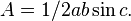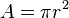# Area facts for kids

Kids Encyclopedia Facts

Area is the amount of space a two dimensional (flat) surface takes up. It is useful because it is how much of a material is needed to make a hollow container; for example, how much wood is needed to make a wardrobe.

You can use different formulas to find the area of different shapes.

• Area of a rectangle is the length of any two touching sides multiplied together. In other words, length times width.
• Area of a triangle is half of the base multiplied by the perpendicular height. This can be found using the trigonometry formula,$A = 1/2 ab \sin c.$.
• Area of a circle:$A = \pi r^2$.

The area of a flat object is related to the surface area and volume of a three-dimensional object.

The area under a curve can be found using integration, from calculus.

Some units used to measure area are square mile and square kilometre.

## Images for kidsArea Facts for Kids. Kiddle Encyclopedia.